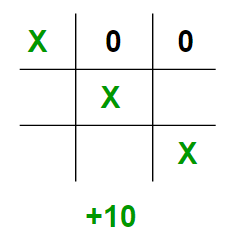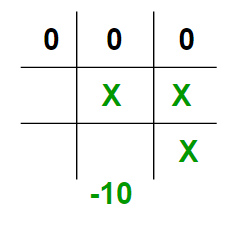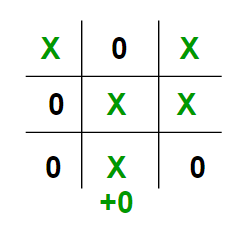# Minimax Algorithm in Game Theory | Set 2 (Introduction to Evaluation Function)

• Difficulty Level : Easy
• Last Updated : 17 Mar, 2022

Prerequisite : Minimax Algorithm in Game Theory
As seen in the above article, each leaf node had a value associated with it. We had stored this value in an array. But in the real world when we are creating a program to play Tic-Tac-Toe, Chess, Backgammon, etc. we need to implement a function that calculates the value of the board depending on the placement of pieces on the board. This function is often known as Evaluation Function. It is sometimes also called Heuristic Function.
The evaluation function is unique for every type of game. In this post, evaluation function for the game Tic-Tac-Toe is discussed. The basic idea behind the evaluation function is to give a high value for a board if maximizer‘s turn or a low value for the board if minimizer‘s turn.
For this scenario let us consider X as the maximizer and O as the minimizer.
Let us build our evaluation function :

1. If X wins on the board we give it a positive value of +10.1. If O wins on the board we give it a negative value of -10.1. If no one has won or the game results in a draw then we give a value of +0.We could have chosen any positive / negative value other than 10. For the sake of simplicity we chose 10 for the sake of simplicity we shall use lower case ‘x’ and lower case ‘o’ to represent the players and an underscore ‘_’ to represent a blank space on the board.
If we represent our board as a 3×3 2D character matrix, like char board; then we have to check each row, each column and the diagonals to check if either of the players have gotten 3 in a row.

## C++

 `// C++ program to compute evaluation function for``// Tic Tac Toe Game.``#include``#include``using` `namespace` `std;` `// Returns a value based on who is winning``// b is the Tic-Tac-Toe board``int` `evaluate(``char` `b)``{``    ``// Checking for Rows for X or O victory.``    ``for` `(``int` `row = 0; row<3; row++)``    ``{``        ``if` `(b[row]==b[row] && b[row]==b[row])``        ``{``            ``if` `(b[row]==``'x'``)``               ``return` `+10;``            ``else` `if` `(b[row]==``'o'``)``               ``return` `-10;``        ``}``    ``}` `    ``// Checking for Columns for X or O victory.``    ``for` `(``int` `col = 0; col<3; col++)``    ``{``        ``if` `(b[col]==b[col] && b[col]==b[col])``        ``{``            ``if` `(b[col]==``'x'``)``                ``return` `+10;``            ``else` `if` `(b[col]==``'o'``)``                ``return` `-10;``        ``}``    ``}` `    ``// Checking for Diagonals for X or O victory.``    ``if` `(b==b && b==b)``    ``{``        ``if` `(b==``'x'``)``            ``return` `+10;``        ``else` `if` `(b==``'o'``)``            ``return` `-10;``    ``}``    ``if` `(b==b && b==b)``    ``{``        ``if` `(b==``'x'``)``            ``return` `+10;``        ``else` `if` `(b==``'o'``)``            ``return` `-10;``    ``}` `    ``// Else if none of them have won then return 0``    ``return` `0;``}` `// Driver code``int` `main()``{``    ``char` `board =``    ``{``        ``{ ``'x'``, ``'_'``, ``'o'``},``        ``{ ``'_'``, ``'x'``, ``'o'``},``        ``{ ``'_'``, ``'_'``, ``'x'``}``    ``};` `    ``int` `value = evaluate(board);``    ``printf``(``"The value of this board is %d\n"``, value);``    ``return` `0;``}`

## Java

 `// Java program to compute evaluation function for``// Tic Tac Toe Game.` `class` `GFG``{` `// Returns a value based on who is winning``// b is the Tic-Tac-Toe board``static` `int` `evaluate(``char` `b[][])``{``    ``// Checking for Rows for X or O victory.``    ``for` `(``int` `row = ``0``; row < ``3``; row++)``    ``{``        ``if` `(b[row][``0``] == b[row][``1``] && b[row][``1``] == b[row][``2``])``        ``{``            ``if` `(b[row][``0``] == ``'x'``)``            ``return` `+``10``;``            ``else` `if` `(b[row][``0``] == ``'o'``)``            ``return` `-``10``;``        ``}``    ``}` `    ``// Checking for Columns for X or O victory.``    ``for` `(``int` `col = ``0``; col < ``3``; col++)``    ``{``        ``if` `(b[``0``][col] == b[``1``][col] && b[``1``][col] == b[``2``][col])``        ``{``            ``if` `(b[``0``][col] == ``'x'``)``                ``return` `+``10``;``            ``else` `if` `(b[``0``][col] == ``'o'``)``                ``return` `-``10``;``        ``}``    ``}` `    ``// Checking for Diagonals for X or O victory.``    ``if` `(b[``0``][``0``] == b[``1``][``1``] && b[``1``][``1``] == b[``2``][``2``])``    ``{``        ``if` `(b[``0``][``0``] == ``'x'``)``            ``return` `+``10``;``        ``else` `if` `(b[``0``][``0``] == ``'o'``)``            ``return` `-``10``;``    ``}``    ``if` `(b[``0``][``2``] == b[``1``][``1``] && b[``1``][``1``] == b[``2``][``0``])``    ``{``        ``if` `(b[``0``][``2``] == ``'x'``)``            ``return` `+``10``;``        ``else` `if` `(b[``0``][``2``] == ``'o'``)``            ``return` `-``10``;``    ``}` `    ``// Else if none of them have won then return 0``    ``return` `0``;``}` `// Driver code``public` `static` `void` `main(String[] args)``{``    ``char` `board[][] =``    ``{``        ``{ ``'x'``, ``'_'``, ``'o'``},``        ``{ ``'_'``, ``'x'``, ``'o'``},``        ``{ ``'_'``, ``'_'``, ``'x'``}``    ``};` `    ``int` `value = evaluate(board);``    ``System.out.printf(``"The value of this board is %d\n"``, value);``}``}` `// This code is contributed by PrinciRaj1992`

## Python3

 `# Python3 program to compute evaluation``# function for Tic Tac Toe Game.` `# Returns a value based on who is winning``# b is the Tic-Tac-Toe board``def` `evaluate(b):`` ` `    ``# Checking for Rows for X or O victory.``    ``for` `row ``in` `range``(``0``, ``3``):``     ` `        ``if` `b[row][``0``] ``=``=` `b[row][``1``] ``and` `b[row][``1``] ``=``=` `b[row][``2``]:``         ` `            ``if` `b[row][``0``] ``=``=` `'x'``:``                ``return` `10``            ``else` `if` `b[row][``0``] ``=``=` `'o'``:``                ``return` `-``10` `    ``# Checking for Columns for X or O victory.``    ``for` `col ``in` `range``(``0``, ``3``):``     ` `        ``if` `b[``0``][col] ``=``=` `b[``1``][col] ``and` `b[``1``][col] ``=``=` `b[``2``][col]:``         ` `            ``if` `b[``0``][col]``=``=``'x'``:``                ``return` `10``            ``else` `if` `b[``0``][col] ``=``=` `'o'``:``                ``return` `-``10` `    ``# Checking for Diagonals for X or O victory.``    ``if` `b[``0``][``0``] ``=``=` `b[``1``][``1``] ``and` `b[``1``][``1``] ``=``=` `b[``2``][``2``]:``     ` `        ``if` `b[``0``][``0``] ``=``=` `'x'``:``            ``return` `10``        ``else` `if` `b[``0``][``0``] ``=``=` `'o'``:``            ``return` `-``10``     ` `    ``if` `b[``0``][``2``] ``=``=` `b[``1``][``1``] ``and` `b[``1``][``1``] ``=``=` `b[``2``][``0``]:``     ` `        ``if` `b[``0``][``2``] ``=``=` `'x'``:``            ``return` `10``        ``else` `if` `b[``0``][``2``] ``=``=` `'o'``:``            ``return` `-``10``     ` `    ``# Else if none of them have won then return 0``    ``return` `0`` ` `# Driver code``if` `__name__ ``=``=` `"__main__"``:`` ` `    ``board ``=` `[[``'x'``, ``'_'``, ``'o'``],``             ``[``'_'``, ``'x'``, ``'o'``],``             ``[``'_'``, ``'_'``, ``'x'``]]``     ` `    ``value ``=` `evaluate(board)``    ``print``(``"The value of this board is"``, value)` `# This code is contributed by Rituraj Jain`

## C#

 `// C# program to compute evaluation function for``// Tic Tac Toe Game.``using` `System;` `class` `GFG``{` `// Returns a value based on who is winning``// b[3,3] is the Tic-Tac-Toe board``static` `int` `evaluate(``char` `[,]b)``{``    ``// Checking for Rows for X or O victory.``    ``for` `(``int` `row = 0; row < 3; row++)``    ``{``        ``if` `(b[row, 0] == b[row, 1] && b[row, 1] == b[row, 2])``        ``{``            ``if` `(b[row, 0] == ``'x'``)``            ``return` `+10;``            ``else` `if` `(b[row, 0] == ``'o'``)``            ``return` `-10;``        ``}``    ``}` `    ``// Checking for Columns for X or O victory.``    ``for` `(``int` `col = 0; col < 3; col++)``    ``{``        ``if` `(b[0, col] == b[1, col] && b[1, col] == b[2, col])``        ``{``            ``if` `(b[0, col] == ``'x'``)``                ``return` `+10;``            ``else` `if` `(b[0, col] == ``'o'``)``                ``return` `-10;``        ``}``    ``}` `    ``// Checking for Diagonals for X or O victory.``    ``if` `(b[0, 0] == b[1, 1] && b[1, 1] == b[2, 2])``    ``{``        ``if` `(b[0, 0] == ``'x'``)``            ``return` `+10;``        ``else` `if` `(b[0, 0] == ``'o'``)``            ``return` `-10;``    ``}``    ``if` `(b[0, 2] == b[1, 1] && b[1, 1] == b[2, 0])``    ``{``        ``if` `(b[0, 2] == ``'x'``)``            ``return` `+10;``        ``else` `if` `(b[0, 2] == ``'o'``)``            ``return` `-10;``    ``}` `    ``// Else if none of them have won then return 0``    ``return` `0;``}` `// Driver code``public` `static` `void` `Main(String[] args)``{``    ``char` `[,]board =``    ``{``        ``{ ``'x'``, ``'_'``, ``'o'``},``        ``{ ``'_'``, ``'x'``, ``'o'``},``        ``{ ``'_'``, ``'_'``, ``'x'``}``    ``};` `    ``int` `value = evaluate(board);``    ``Console.Write(``"The value of this board is {0}\n"``, value);``}``}` `// This code is contributed by Rajput-Ji`

## Javascript

 ``

Output :

`The value of this board is 10`

The idea of this article is to understand how to write a simple evaluation function for the game Tic-Tac-Toe. In the next article we shall see how to combine this evaluation function with the minimax function. Stay Tuned.